Geomery section of MathBitsNotebook.comPractice Test 1 - CCSS Geometry Interactive Version - Long ResponseDirections:  Questions for Parts II, III, and IV are long response questions. You will need paper, pencil and a graphing calculator to work out the problems. Check your answer after solving each problem by clicking the ANSWER button.
Part II: (2 pts)
 25. Given circle O with diameter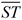and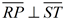. PT is 12 less than SP and RP = 8. Find PT.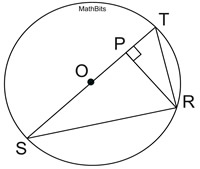26. ΔABC is a dilation of ΔDEF by a scale factor of 3. If m∠E = 90º, DE = 5 and DF =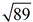, express the sin(∠A) as a fraction.
 27. A cube of cheese has side lengths of 8 inches. Using a cheese cutter, the cube is sliced into two sections with a cut along the diagonal from one side, as shown. Find the area of the cross section formed by the slice.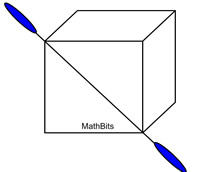28. You are given three clues to find a specific point on a coordinate axis. Start at the point (4,3). Clue 1: move to the image after the translation (x,y) → (x - 8,y + 4) Clue 2: now, reflect your position over the x-axis. Clue 3: now, rotate your position 90º counterclockwise. What are the coordinates of your final positon?
 29. Given the diagram shown at the right, find the value of 3x.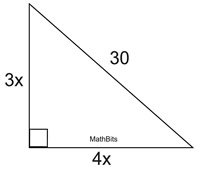30. Does the circle with a center at (2,3) and passing through the point (8,9), also pass through the point (-4,-1)? Explain your answer.
 31. Jonathan incorrectly states that the sum of the measures of the exterior angles of a triangle is 180º. Draw an example and explain to Jonathan how to correctly find the sum of the measures of the exterior angles of a triangle.

Part III:
(4 pts)
 32. In the diagram at the right, C lies on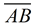. If the ratio of AC to CB is 3 : 2, what is the y-coordinate of C?33. A squirrel is sitting atop a roof looking at a nearby tree. The horizontal distance from the squirrel to the tree (A) is 35 feet. The angle of elevation of the top (B) of the tree is 28º and the angle of depression of the foot (C) of the tree is 37º. What is the height of the tree, to the nearest tenth of a foot ?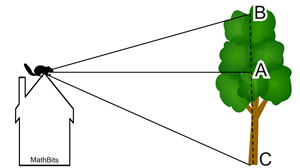34. Given parallelogram ABCD, m∠BAD = 56º, m∠ABC = (8a - b)º and m∠BCD = (2a + 6b)º. Find the values of a and b.

Part IV:
(6 pts)
 35. The coordinates of quadrilateral ABCD are located at A(-2,3), B(4,5), C(6,-1) and D(0,-3). a) Using coordinate geometry methods, show that the diagonals bisect each other. b) Using coordinate geometry methods, show that the diagonals are perpendicualr. c) Using coordiante geometry methods, show that all four sides are congruent. d) Based only upon the information shown in parts a, b and c, what name can be given to this quadrilateral?
 36. Sam's Pizza Shop uses open-topped cardboard boxes to serve full circular pizzas to the seated customers. These serving boxes come in three sizes.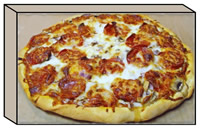a) The open topped serving boxes are cut from a square sheet of cardboard by cutting out squares with 2 inch sides from each corner and then folding up the sides. If the volume of a "large pizza" serving box is 648 cubic inches, state the dimensions (length, width, height) of the serving box, in inches. b) When placed in its serving box, a large pizza can be described as being "inscribed" in the box. State the surface area of a large pizza, to the nearest square inch. c) Sam's Pizza Shop offers a special "bacon ribbed" pizza where strips of bacon are placed along the circumference of the pizza. When served, pizzas are cut into 8 slices. How many inches (to the nearest inch) of bacon are needed for each slice of a large bacon ribbed pizza?